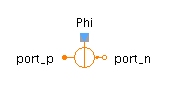Mean - MapleSim Help

Mean

Compute the mean overDescription The Mean component computes the element-wise mean over the period of a specified frequency. The Signal Size parameter assigns the dimension of the input and output connectors.Equations ${y}_{j}=\left\{\begin{array}{cc}0& t<{t}_{0}+\frac{1}{f}\\ f{\int }_{T}^{T+\frac{1}{f}}{u}_{j}\mathrm{dt}& \mathrm{otherwise}\end{array}$ $T={t}_{0}+\frac{\left|\left(t-{t}_{0}\right)f\right|-1}{f}$ $\mathrm{with}{t}_{0}\mathrm{the start-time of the simulation}$Connections

 Name Description Modelica ID $u$ Real input vector u $y$ Real output vector yParameters

 Name Default Units Description Modelica ID $f$ $50$ $\mathrm{Hz}$ Base frequency f Signal Size $1$ Dimension of input and output signals signalSizeModelica Standard Library The component described in this topic is from the Modelica Standard Library. To view the original documentation, which includes author and copyright information, click here.